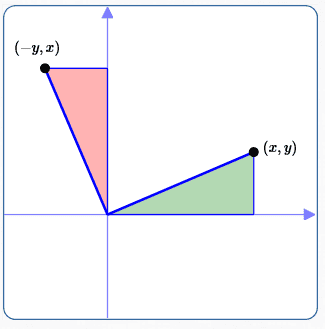﻿ Two Useful Transforms: Reflection About the Line y = x, and Counterclockwise Rotation by 90 Degrees

# Two Useful Transforms: Reflection About the Line $\,y = x\,$ and Counterclockwise Rotation by $\,90^\circ$

• PRACTICE (online exercises and printable worksheets)

This section is optional in the Precalculus course.

Once we have the ellipse equation for foci on the $x$-axis $\displaystyle\,\left(\frac{x^2}{a^2} +\frac{y^2}{b^2} = 1\right)\,,$
we don't have to go through that long and tedious derivation a second time to get the equation for foci on the $y$-axis.
There are easier ways!

If desired, we can use appropriate transforms to get the new graph.
This section discusses two transforms that can be used.

## Reflection About the Line $\,y = x\,$

• As discussed in an earlier section, reflection about the line $\,y = x\,$ switches the coordinates of a point:
$\,(x,y)\,$ moves to $\,(y,x)\,.$
• For example, suppose that reflection about $\,y = x\,$ is applied to the ellipse $\displaystyle\,\frac{x^2}{a^2} +\frac{y^2}{b^2} = 1\,,$
which has its foci on the $x$-axis.
Then
• $\,(c,0)\,$ moves to $\,(0,c)\,$
• $\,(-c,0)\,$ moves to $\,(0,-c)\,$
So, the reflected ellipse has its foci on the $y$-axis.
• In an equation, reflection about the line $\,y = x\,$ is accomplished by switching the variables $\,x\,$ and $\,y\,.$
• For example, switching $\,x\,$ and $\,y\,$ turns $$\frac{x^2}{a^2} +\frac{y^2}{b^2} = 1$$ into: $$\frac{y^2}{a^2} +\frac{x^2}{b^2} = 1$$

As you'll see in the next section, this new equation is precisely
the ellipse with center at the origin and foci along the $y$-axis!
So easy!

## Counterclockwise Rotation by $\,90^\circ$

 As illustrated by the sketch at right, rotating counterclockwise by $\,90^\circ\,$ moves $\,(x,y)\,$ to $\,(-y,x)\,.$ Think of the green triangle as a wedge of wood; grab it and rotate it, so the side on the $x$-axis moves to the $y$-axis. It ‘turns into’ the red triangle! How can an equation be ‘adjusted’ to accomplish this $\,90^\circ\,$ counterclockwise rotation? Be very careful! Since $\,(x,y)\,$ moves to $\,(-y,x)\,,$ you might be tempted to think that, in the original equation, you should replace every $\,x\,$ by $\,-y\,$ and every $\,y\,$ by $\,x\,.$ But, THIS IS WRONG!Here are the correct replacements in an equation,
to rotate its graph counterclockwise by $\,90^\circ\,$:
• replace every $\,x\,$ by $\,y\,$
• replace every $\,y\,$ by $\,-x\,$

For example, applying a $\,90^\circ\,$ counterclockwise rotation to the equation $\,y = \sqrt{x}\,$ gives the following: $$\begin{gather} \overbrace{y}^{\text{replace by \,-x\,}} = \sqrt{\overbrace{x}^{\text{replace by \,y\,}}}\cr\cr -x = \sqrt{\vphantom{h}\, y\, } \end{gather}$$
 Look at the plot results at right from WolframAlpha. The equation $\,y = \sqrt x\,$ is plotted in blue. The equation $\,-x = \sqrt y\,$ is plotted in purple. Notice the beautiful $\,90^\circ\,$ counterclockwise rotation (from the blue curve to the purple curve), as desired!## So, what's going on here?

In a graph, we want $\,(x,y)\,$ to move to $\,(-y,x)\,.$
In the equation, this is accomplished by:

• replacing every $\,x\,$ by $\,y\,$
• replacing every $\,y\,$ by $\,-x\,$
Why does it work this way?
What's going on here?

Here's the correct thought process:

• Every equation in two variables ($\,x\,$ and $\,y\,$) can be written in the form $\,f(x,y) = 0\,.$
For example, the equation $\,y = x^2\,$ can be written as $\,y - x^2 = 0\,,$ so defining $\,f(x,y) := y - x^2\,$ does the job.
• The graph of the original equation, $\,f(x,y) = 0\,,$ is (by definition) $$\{\ (x,y) \ \ |\ \ f(x,y) = 0\ \} \qquad (*)$$ Read (*) as:   the set of all points $\,(x,y)\,$ with the property that $\,f(x,y) = 0\,.$
• The set of points we want, after rotating the points in (*) counterclockwise by $\,90^\circ\,,$ is: $$\{\ (-y,x) \ \ |\ \ f(x,y) = 0\ \} \qquad (**)$$
• Define $\,\hat x := -y\,$ and $\,\hat y := x\,,$ to view the points in (**) in a more natural way: $$\{\ (\ \overbrace{\strut -y}^{\hat x}\ ,\ \overbrace{\strut x}^{\hat y}\ ) \ \ |\ \ f(x,y) = 0\ \} \qquad (**)$$ (Read $\,\hat x\,$ as ‘$x$ hat’ and $\,\hat y\,$ as ‘$y$ hat’.)
• Solve for $\,x\,$ and $\,y\,$ in terms of $\,\hat x\,$ and $\,\hat y\,$:
• $\,x = \hat y\,$
• $\,y = -\hat x\,$
• Rename (**): \begin{align} \{(\overbrace{\strut -y}^{\hat x}&,\overbrace{\strut x}^{\hat y}) \ \ |\ \ f(x,y) = 0\}\cr\cr &= \{(\hat x,\hat y)\ \ |\ \ f(\hat y,-\hat x) = 0\}\cr\cr &= \{(x,y)\ \ |\ \ f(y,-x) = 0\}\qquad \qquad \text{(return to the dummy variables \,x\, and \,y\,)} \end{align}
• How do we get from the original equation ‘$\,f(x,y) = 0\,$’ to the new (desired) equation ‘$\,f(y,-x) = 0\,$’?
Answer: Replace every $\,x\,$ by $\,y\,,$ and replace every $\,y\,$ by $\,-x\,$!

EXAMPLE
Rotate the ellipse $\displaystyle\,\frac{x^2}{a^2} +\frac{y^2}{b^2} = 1\,$ counterclockwise by $\,90^\circ\,$: $$\begin{gather} \frac{y^2}{a^2} +\frac{(-x)^2}{b^2} = 1\cr\cr \frac{y^2}{a^2} + \frac{x^2}{b^2} = 1 \end{gather}$$ As before, you'll see in the next section that this is precisely
the ellipse with center at the origin and foci along the $y$-axis!

Master the ideas from this section
equations of ellipses in standard form: foci on the $\,y$-axis